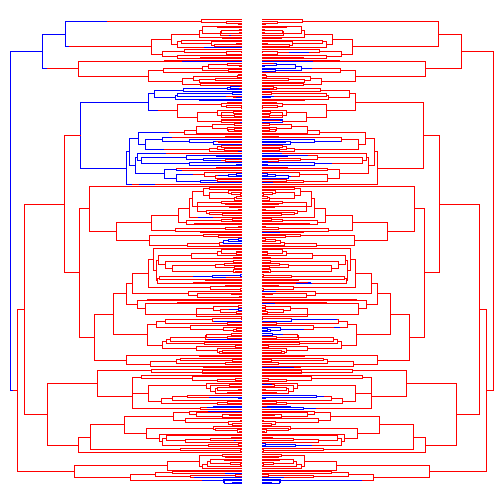## Thursday, December 4, 2014

### Simulating correlated evolution of discrete characters under Pagel's model

In a recent post, in which I gave an R function to run Pagel's (1994) test for correlation between two binary traits, I also posted (without much comment) some simple code to simulate the character histories of binary traits undergoing correlated evolution by Pagel's model.

Here is that code again with some explanation.

(1) First, just as a preliminary, let's load our packages & simulate a stochastic phylogeny:

``````library(phytools)
``````
``````## Loading required package: ape
``````
``````tree<-pbtree(n=300,scale=1)
``````

(2) Next, we need to set up a matrix to simultaneously simulate our two traits. Remember, under Pagel's model, character correlation are just transition rates in one character that depend on the state of a second. To simulate under this model we actually have to simultaneously simulate states for the two characters at once. Here, as I did last time, I'll keep it simple. Let's say that the two characters have states `a` and `b`. I'll let the transition rates into states `a,a` or `b,b` be high (say `2.0`), but I will make the transition rates to any state `a,b` or `b,a` low - say 0.4. This is what that looks like:

``````Q<-matrix(c(0,0.4,0.4,0,2,0,0,2,2,0,0,2,0,0.4,0.4,0),4,4,byrow=TRUE)
rownames(Q)<-colnames(Q)<-c("aa","ab","ba","bb")
diag(Q)<--rowSums(Q)
Q
``````
``````##      aa   ab   ba   bb
## aa -0.8  0.4  0.4  0.0
## ab  2.0 -4.0  0.0  2.0
## ba  2.0  0.0 -4.0  2.0
## bb  0.0  0.4  0.4 -0.8
``````

(3) Now, we can simulate both characters simultaneously up the tree using the phytools function `sim.history`.

``````tt<-sim.history(tree,Q)
``````
``````## Note - the rate of substitution from i->j should be given by Q[j,i].
## Detecting that rows, not columns, of Q sum to zero :
##   Transposing Q for internal calculations.
## Done simulation(s).
``````

(4) This is the history of our two character state data; however we still have to backtranslate this into the character histories for each of our two traits. To do this, we can use `mergeMappedStates` to merge `a,a` and `a,b` (for instance) into `a` for character 1 and so on. Here is what that looks like:

``````t1<-mergeMappedStates(tt,c("aa","ab"),"a")
t1<-mergeMappedStates(t1,c("ba","bb"),"b")
t2<-mergeMappedStates(tt,c("aa","ba"),"a")
t2<-mergeMappedStates(t2,c("ab","bb"),"b")
t1\$states<-getStates(t1,"tips")
t2\$states<-getStates(t2,"tips")
``````

(5) For fun, let's plot the two histories (just as we did last time) so that we can see that they are indeed highly correlated:

``````par(mfrow=c(1,2))
plotSimmap(t1,setNames(c("red","blue"),letters[1:2]),lwd=1,ftype="off")
plotSimmap(t2,setNames(c("red","blue"),letters[1:2]),lwd=1,ftype="off",
direction="leftwards")
``````(6) Finally, we can fit the Pagel (1994) model:

``````x<-getStates(t1,"tips")
y<-getStates(t2,"tips")
source("fitPagel.R")
.check.pkg<-phytools:::.check.pkg
fit<-fitPagel(tree,x,y)
fit
``````
``````##
##   Pagel's binary character correlation test:
##
## Indepedent model rate matrix:
##            a|a        a|b        b|a        b|b
## a|a -0.9753616  0.5606835  0.4146781  0.0000000
## a|b  2.7557800 -3.1704581  0.0000000  0.4146781
## b|a  2.7479439  0.0000000 -3.3086275  0.5606835
## b|b  0.0000000  2.7479439  2.7557800 -5.5037240
##
## Dependent model rate matrix:
##            a|a        a|b        b|a       b|b
## a|a -0.7760794  0.4795664  0.2965130  0.000000
## a|b  5.1666938 -7.2422057  0.0000000  2.075512
## b|a  2.0876921  0.0000000 -4.0078768  1.920185
## b|b  0.0000000  3.8020580  0.7652618 -4.567320
##
## Model fit:
##             log-likelihood
## independent      -186.3963
## dependent        -173.9505
##
## Hypothesis test result:
##   likelihood-ratio:  24.89146
##   p-value:  5.290212e-05
``````

That's it. Hopefully this is helpful to someone!